# Binary Search : Analysis

### Binary Search : Space/Time Complexity Analysis of Binary Search

Binary search is one of the searching algorithms which falls into the category of divide and conquer algorithms. It is used to search an element in an array/vector in efficient time i.e. O(logn) time complexity.

Examples:

```Example 1:
Input: arr[]=2,4,6,10,12 target_element=10;
Output: Found

Example 2:
Input: arr[]=1,4,6,12,99 target_element=10;
```

### Intuition:

Divide and conquer algorithms have 3 steps:

1. Divide: Divide the problem into subproblems and those sub-problems into further sub-problems and so on.
2. Conquer: Solve those sub-problems.
3. Combine: Combine the solutions of sub-problems to get the solution of the main problem.

Binary search is one of those algorithms which uses this approach and helps in finding the target element.

Read about Binary Search in details here

## Steps:

1. We divide the sorted array into two parts by calculating the middle index.
2. We compare the element at the middle index with the target element. If it matches we return TRUE/index of that element.
3. If it doesn’t match, we check if it’s greater than the middle element or smaller.
4. If it’s smaller than middle element, then we reduce our search space to right of middle index [0 to middle-1] else if its greater than middle element, we reduce our search space to left of middle index [m+1 to n-1 (n: size of array/vector)]
5. We again find the middle index for that search space and follow step number 2,3,4.
6. If the element is present in the array, it will always return TRUE/index of that element or FALSE.

The following diagram explains the above approach

### The middle index can be calculated using the following formula: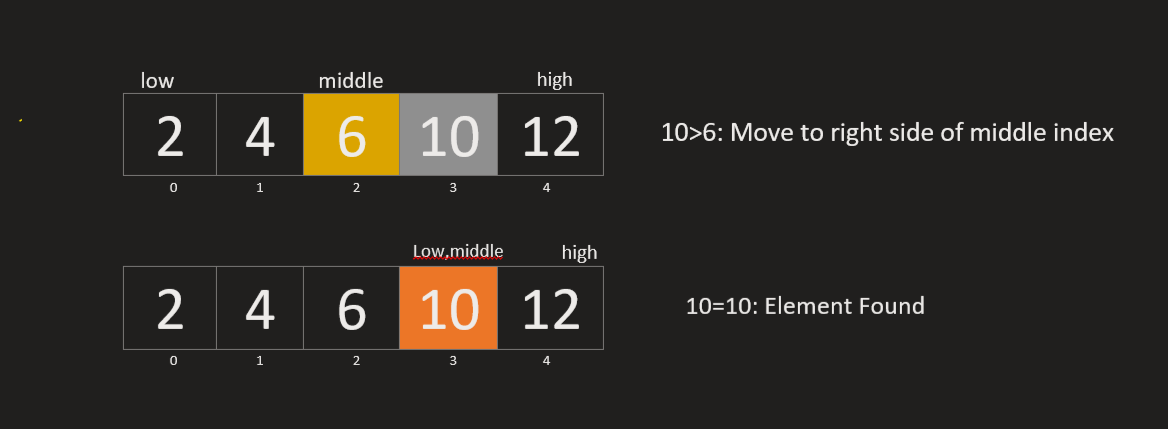Another diagram gives a better understanding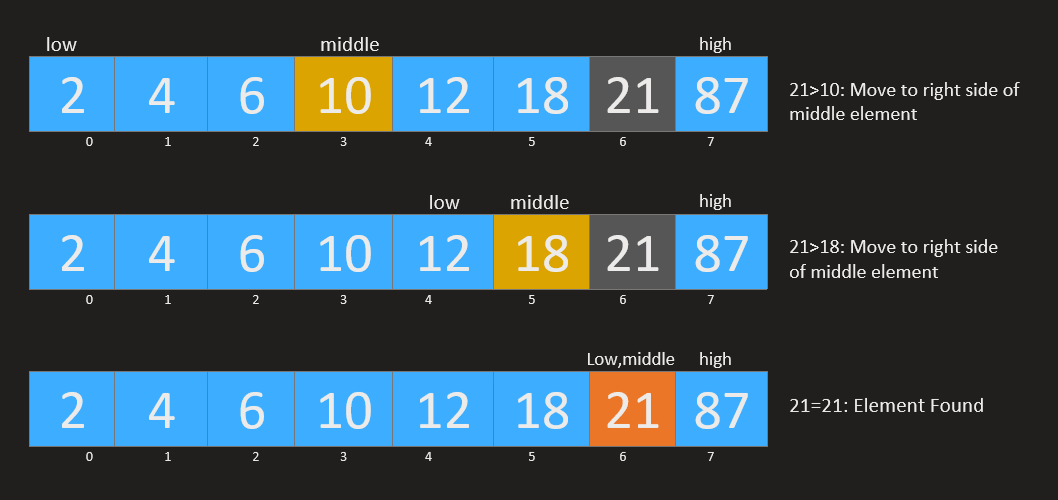### Time Complexity of Binary Search

Looking at the recursive code of binary search, we can write the recurrence relation for binary search in the form: Time is taken by the control statements+time taken by the recursive call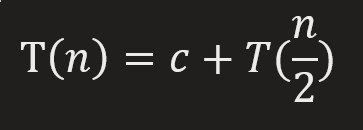……………………………equation 1

Now if we find the recurrence equation for searching in n/2 elements in the array, we can substitute n/2 in the given relation which gives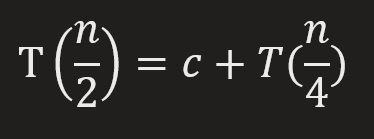…………………………..equation 2

Substituting the above equation in equation 1 gives: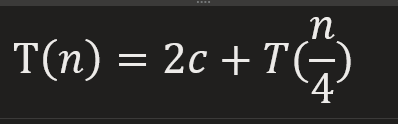………………………..equation 3

Now we find the recurrent equation for searching in n/4 elements in the array, we can substitute n/4 in equation 1, which gives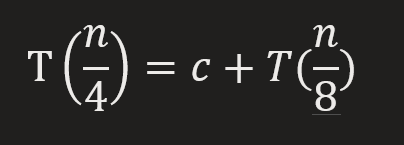…………………………equation 4

Again substituting this in equation 3, we get,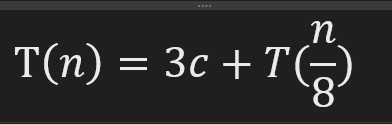……………………………..equation 5

From here we can find the general equation of T(n)………………………………equation 6

At some point, we can see that the second term in the equation would almost become equal to 1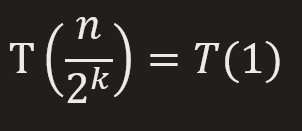From here we calculate the value of k which is equal to log n and substituting this value in equation 6, we get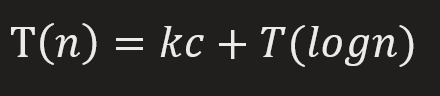To represent this in O notation, we ignore the constant terms and we get O(logn) as our time complexity

## C++ Code

``````#include<bits/stdc++.h>
using namespace std;

bool bin_search_recursive(int arr[], int target, int low, int high) {
if (low > high) return false;
int mid = (low + high) / 2;
if (arr[mid] == target) return true;
else if (arr[mid] > target) bin_search_recursive(arr, target, low, mid - 1);
else bin_search_recursive(arr, target, mid + 1, high);
}
int main() {
int arr[] = {2,4,6,10,12};
int size = 5;
int target = 10;
bin_search_recursive(arr, target, 0, size - 1) ? cout << "Element found" :
}
``````

Output: Element found

Time Complexity: O(logn)

Space Complexity: O(1)

## Java Code

``````class bin_search {
static boolean bin_search_recursive(int arr[], int target, int low, int high) {
if (low > high) return false;
int mid = (low + high) / 2;

if (arr[mid] == target) return true;
else if (arr[mid] > target) return bin_search_recursive(arr, target, low, mid - 1);
else return bin_search_recursive(arr, target, mid + 1, high);
}
public static void main(String[] args) {
int arr[] = {2,4,6,10,12};
int size = 5;
int target = 10;
if (bin_search_recursive(arr, target, 0, size - 1)) {
System.out.println("Element found");
}
}
}
``````

Output: Element found

Time Complexity: O(logn)

Space Complexity: O(1)

## C++ Code

``````#include<bits/stdc++.h>
using namespace std;
bool bin_search_iterative(int arr[], int target, int low, int high) {
while (low <= high) {
int mid = (low + high) / 2;
if (arr[mid] == target) return true;
if (arr[mid] > target) high = mid - 1;
else low = mid + 1;
}
return false;
}
int main() {
int arr[] = {2,4,6,10,12};
int size = 5;
int target = 12;
bin_search_iterative(arr, target, 0, size - 1) ? cout << "Element found" : cout << "Element not found";
}
``````

Output: Element found

Time Complexity: O(logn)

Space Complexity: O(1)

## Java Code

``````class bin_search {
static boolean bin_search_iterative(int arr[], int target, int low, int high) {
while (low <= high) {
int mid = (low + high) / 2;
if (arr[mid] == target) return true;
else if (arr[mid] > target) high = mid - 1;
else low = mid + 1;
}
return false;
}
public static void main(String[] args) {
int arr[] = {2,4,6,10,12};
int size = 5;
int target = 10;
if (bin_search_iterative(arr, target, 0, size - 1)) {
System.out.println("Element found");